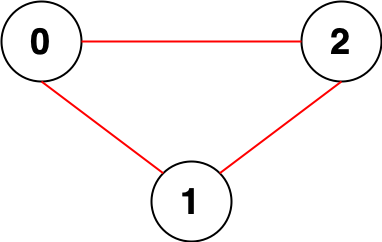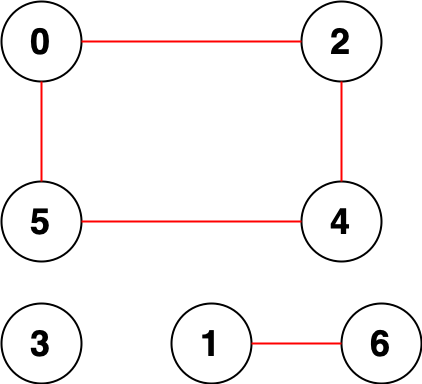# 战局详情

640 / 3847Juruoer130:39:050:02:510:20:140:39:05

# 题目及解答

2315. 统计星号

• 1 <= s.length <= 1000
• s 只包含小写英文字母，竖线 '|' 和星号 '*'
• s 包含 偶数 个竖线 '|'

2316. 统计无向图中无法互相到达点对数

• 1 <= n <= 1e5
• 0 <= edges.length <= 2 * 1e5
• edges[i].length == 2
• 0 <= ai, bi < n
• ai != bi
• 不会有重复边。[[0,1],[0,3],[0,6],[1,2],[1,3],[1,4],[1,5],[2,3],[2,6],[3,4],[3,5],[3,6],[4,6],[5,6]]

2317. 操作后的最大异或和

• 1 <= nums.length <= 1e5
• 0 <= nums[i] <= 1e8

• 对于一个已有的非负整数 num任意一个 期望获得的非负整数 target ，我们都可以选择出一个非负整数 x ，使得 num XOR x = target
• 对于一个已有的非负整数 num ，对于 num 的二进制中 任意一个1 的位 idx，我们都可以选择出一个非负整数 x，进行操作 num = num AND x 后， num 的二进制中第 idx 位的 1 变为 0 ，而不改变 num 的二进制中其他位的值。

2318. 不同骰子序列的数目

1. 序列中任意 相邻 数字的 最大公约数1
2. 序列中 相等 的值之间，至少有 2 个其他值的数字。正式地，如果第 i 次掷骰子的值 等于j 次的值，那么 abs(i - j) > 2

• 1 <= n <= 1e4

(1, 2, 1, 3) 是不可行的，因为第一个和第三个骰子值相等且 abs(1 - 3) = 2 （下标从 1 开始表示）。
(1, 2, 3, 6) i是不可行的，因为 3 和 6 的最大公约数是 3 。

dp[k][i][j] 表示直到第 k 次投骰子，投到的是 i，且前一次投骰子投到的是 j 的序列数，且仅当 ij 互质时才有意义

• k == 2 时，在 k = 1 次投骰子结果确定的情况下，仅有一种可能 (感觉在说废话)dp[i][j] = 1ji 互质）。

• k > 2 时，需要取出第 k - 1 次投骰子结果为 j 且第 k - 2 次投骰子结果与 i 不同是所有结果， dp[k][i][j] = Σ(dp[k - 1][j][r])r16ij 互质，jr 互质，i != r